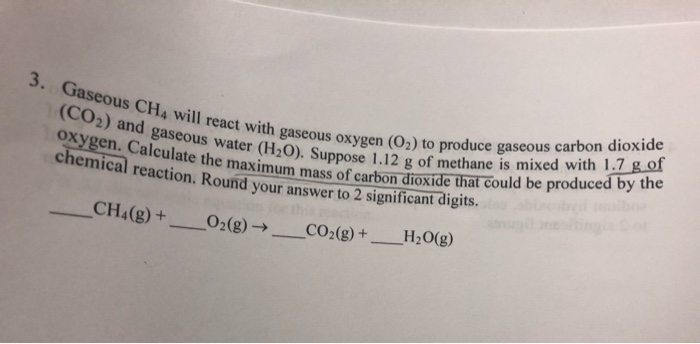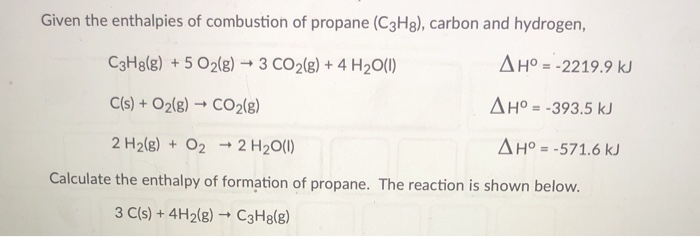Question

Calculate the amount of carbon dioxide produced when burning 10.0 g of propane with 5 g of oxygen in the following reaction.

C3H8      +      5 O2     ------->      3 CO2       +      4 H2O

Let us first calculate the number of moles of O2 and propane used in the reaction. The molar mass of propane and O2 are, 44.1 g/mol and 32 g/ mol, respectively. The moles of propane and O2 can be calculated using following formula

Moles = weight/ molar mass

Moles of propane = 10 g/ 44.1 g/mol = 0.227 mol

Moles of O2 = 5 g / 32 g/mol = 0.156 mol

From the balanced chemical equation it is clear that 1 mol of propane requires 5 moles of O2. Therefore, we can consider

1 mol propane = 5 mol of O2

For. 0.227 mol propane = 0.227 x 5 = 1.135 mol of O2

Thus for 0.227 mol of propane 1.135 mol of O2 are required. However, actual number of moles of O2 used are 0.156 mol. Therefore, propane is used in excess while O2 is the limiting reagent as it getting consumed completely. Therefore, the yield of carbon dioxide will depend on the number of moles of O2 used in the reaction.

From the balanced chemical equation it is clear that 5 moles of O2 produce 3 moles of CO2. Therefore, we can consider

5 moles of O2 = 3 moles of CO2

For. 0.156 moles O2 = (0.156 x 3) / 5 mol of CO2

= 0.094 moles of CO2

The molar mass of CO2 is 44.01 g/mol. The mass of CO2 produced can be calculated using following formula

Grams of CO2 = moles x molar mass

= 0.094 mol x 44.01 g/mol

= 4.12 grams

Therefore, 4.12 grams of CO2 will be produced

#### Earn Coins

Coins can be redeemed for fabulous gifts.

Similar Homework Help Questions
• ### Using the following equation for the combustion of propane, calculate the amount of propane consumed if...

Using the following equation for the combustion of propane, calculate the amount of propane consumed if the reaction gave off 333 kJ heat. C3H8(g) + 5 O2(g) --> 3 CO2(g) + 4 H2O(g) ΔH = -2044 kJ

• ### Write a balanced chemical equation for the reaction for propane C3H8 burning in oxygen to for carbon dioxide and water vapor

Write a balanced chemical equation for the reaction for propane C3H8 burning in oxygen to for carbon dioxide and water vapor

• ### If you start with #7.0# moles of propane and #7.0# moles of oxygen gas what is the percent yield if #4.0# moles of carbon dioxide are produced?

Consider the reaction below. If you start with 7.0 moles of C3H8 (propane) and 7.0 moles of O2, _ is the percent yield if 4.0 moles of carbon dioxide is produced. C3H8(g) + 5O2(g) --> 3CO2(g) + 4H2O(g)

• ### 1.Calculate the amount of heat in joules that is produced by burning 1.0 pound of propane....

1.Calculate the amount of heat in joules that is produced by burning 1.0 pound of propane. delta Hf naught: (C3H8) = -103.85 kJ/mole

• ### Liquid hexane (CH3(CH2)4CH3) will react with gaseous oxygen (O2) to produce gaseous carbon dioxide (CO2) and gaseous water(H2O). Suppose 5.2 g of hexane is mixed with 23.7 g of oxygen. Calculate the maximum mass of carbon dioxide that could be produced

Liquid hexane  (CH3(CH2)4CH3) will react with gaseous oxygen (O2) to produce gaseous carbon dioxide (CO2)  and gaseous water(H2O). Suppose 5.2 g of hexane is mixed with 23.7 g of oxygen. Calculate the maximum mass of carbon dioxide that could be produced by the chemical reaction. Round your answer to  significant digits.

• ### 8. When glucose reacts with oxygen in living systems, carbon dioxide and water are produced, and...

8. When glucose reacts with oxygen in living systems, carbon dioxide and water are produced, and a great deal of energy is liberated. C6H12O6(s) + 6 O2(g)  6 CO2(g) + 6 H2O(g) What weight of carbon dioxide can be produced from the reaction of 10.0 grams of glucose with 10.0 grams of oxygen? (a) 2.29 g (b) 2.44 g (c) 13.8 g (d) 14.7 g (e) none of these 9.Based on the balanced chemical equation shown below, determine the...

• ### 3. Gaseous CH4 will react with gaseous oxygen (O2) to produce gaseous carbon dioxide (CO2) and...3. Gaseous CH4 will react with gaseous oxygen (O2) to produce gaseous carbon dioxide (CO2) and gaseous water (H20). Suppose 1.12 g of methane is mixed with 1.7 g of Oxygen. Calculate the maximum mass of carbon dioxide that could be produced by the chemical reaction. Round your answer to 2 significant digits. CH4(g)+ 02 (g) ->_CO2(g) + H2O(g) 5. The following chemical reaction takes place in aqueous solutions: 2AGF(aq) + (NH4)2 CO 3 (aq) Ag, CO3 (aq) + 2NH4F(aq)...

• ### Many power plants produce energy by burning carbon-based fuels, which also produces carbon dioxide. Carbon dioxide...

Many power plants produce energy by burning carbon-based fuels, which also produces carbon dioxide. Carbon dioxide is a greenhouse gas, so over-production can have negative effects on the environment. Use enthalpy of formation data to calculate the number of moles of CO2(g) produced per megajoule of heat released from the combustion of each fuel under standard conditions (1 atm and 25 �C). Each must be in mol * MJ^-1 (a) coal, C(s, graphite) (b) natural gas, CH4(g); (c) propane, C3H8(g);...

• ### Given the enthalpies of combustion of propane (C3Hg), carbon and hydrogen, C3H3(g) + 5 O2(g) +...Given the enthalpies of combustion of propane (C3Hg), carbon and hydrogen, C3H3(g) + 5 O2(g) + 3 CO2(g) + 4 H20(1) AH° = -2219.9 kJ C(s) + O2(g) + CO2(8) AH° = -393.5 kJ 2 H2(g) + O2 + 2 H2O(1) AH° = -571.6 kJ Calculate the enthalpy of formation of propane. The reaction is shown below. 3 C(s) + 4H2(g) → C3H3(g)